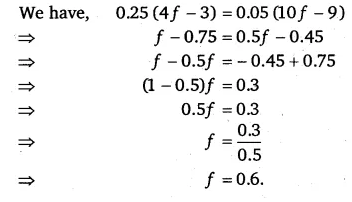# Simplify and solve the following linear equations: 0.25(4f – 3) = 0.05(10f – 9)

Simplify and solve the following linear equations:
0.25(4f – 3) = 0.05(10f – 9).